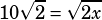Test 1 Section 3 Number 20

This is an easy one to get tied up on! First, double the first equation so that you’ve gotsomething in both equations.Now recognize that the question asks you nothing about a. It only wants you to solve for x! So use the transitive property to set up one equation with one variable:Solve by squaring both sides and then doing a little more algebraic manipulation: